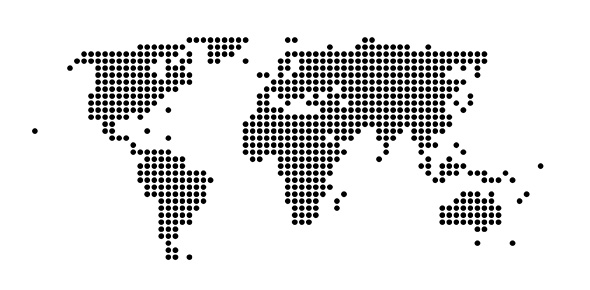# Scale Factor Satterfield

8 QuestionsSettingsScale factor, scale drawings, measurement conversions

Related Topics
• 1.
3 quarts = __________ cups
• A.

3 cups

• B.

6 cups

• C.

8 cups

• D.

12 cups

• 2.
A map has a scale of 1in = 3mi. The distance from Spencer's house to Waffle House is 8in. What is the distance from Spencer's house to Waffle House in miles?
• A.

27 mi

• B.

11 mi

• C.

20 mi

• D.

24 mi

• 3.
The map of Georgia has a scale of 1/2 inches = 12mi. The map shows the distance from Gainesville to Columbus is 4 inches. What is the actual distance from Gainesville to Columbus in miles?
• A.

24 mi

• B.

48 mi

• C.

96 mi

• D.

108 mi

• 4.
A ferris wheel is 72 meters tall. A model of the ferris wheel is 9 centimeters tall. Which is the scale of the model ferris wheel to the actual ferris wheel?
• A.

8 m = 1 cm

• B.

1 cm = 9 m

• C.

1 cm = 8 m

• D.

9 cm = 1 m

• 5.
12 cm                                       36 cmWhat is the scale factor of the two similar figures?
• A.

24 cm

• B.

48 cm

• C.

3 cm

• D.

432 cm

• 6.
Hi                       4 ft                                          12 ft                               3 ftFind the length of the corresponding side.
• A.

4 ft

• B.

1 ft

• C.

0.25 ft

• D.

1/4 ft

• 7.
8 cups = _________pints
• A.

2 pints

• B.

4 pints

• C.

12 pints

• D.

16 pints

• 8.
S 48 feet = ____________ yards
• A.

24 yards

• B.

48 yards

• C.

36 yards

• D.

16 yards